### Monotone and FCS Regression Methods

The regression method is the default imputation method in the MONOTONE and FCS statements for continuous variables.

In the regression method, a regression model is fitted for a continuous variable with the covariates constructed from a set of effects. Based on the fitted regression model, a new regression model is simulated from the posterior predictive distribution of the parameters and is used to impute the missing values for each variable (Rubin, 1987, pp. 166–167). That is, for a continuous variable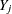with missing values, a model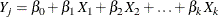is fitted using observations with observed values for the variableand its covariates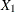,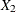, …,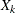.

The fitted model includes the regression parameter estimates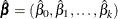and the associated covariance matrix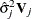, where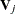is the usualinverse matrix derived from the intercept and covariates,, …,.

The following steps are used to generate imputed values for each imputation:

1. New parameters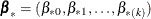and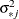are drawn from the posterior predictive distribution of the parameters. That is, they are simulated from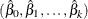,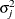, and. The variance is drawn as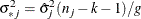where g is a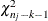random variate and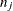is the number of nonmissing observations for. The regression coefficients are drawn as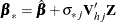where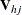is the upper triangular matrix in the Cholesky decomposition,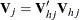, andis a vector of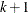independent random normal variates.

2. The missing values are then replaced by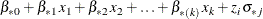where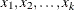are the values of the covariates and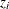is a simulated normal deviate.